# Catch up on the latest AI articles# We Want To Learn Generic Rewards From Human Motion Videos That We Can Use To Train Our Robots!

3 main points
✔️ Learns reward functions with high generalization performance by using diverse and large scale human videos
✔️ Solve tasks using the learned reward function even for unknown tasks and environments
✔️ Demonstrates high success rate in experiments with real robots

ritten by Annie S. Cgeb,Suraj Nair ,Chelsea Finn,
(Submitted on 31 Nar 2021)
Subjects: Robotcis (cs.RO), Artificial Intelligence (cs.AI), Computer Vision and Pattern Recognition (cs.CV), Machine Learning (cs.LG)

The images used in this article are from the paper, the introductory slides, or were created based on them.

## first of all

For a robot to be able to solve various tasks in various environments, it is essential to have indicators and rewards for whether the tasks are successful, which are necessary for planning and reinforcement learning. In particular, in the real world, the reward function must be generic to various tasks, objects, and environments, using information obtained only from onboard sensors such as RGB images. A certain degree of generality has been achieved by using large and diverse datasets in areas such as computer vision and natural language, but not in the field of robotics. However, in the field of robotics, it is costly and difficult to collect such a large and diverse dataset. In this study, we introduce a method called Domain-agnostic Video Discriminator (DVD), which learns a reward function that can cope with an unknown task by making good use of videos of humans solving tasks, such as those available on YouTube. which learns a reward function that can handle unknown tasks. We have shown that this method can estimate rewards for unknown tasks and environments using a relatively large dataset of humans solving tasks and a small amount of data about robots. In the next section, we will explain in detail how the method works.

## technique

In the first place, human data is very different from the observation space of robots, and of course, robots and humans themselves have different shapes. In addition, the action space of humans and robots is also very different, and it is not possible to convert all human actions into robot actions. In addition, the so-called "in-the-wild" data, which can be found on the web, has problems such as different viewpoints and backgrounds, and noise. However, we are still motivated by the fact that we can easily access a lot of data, and we want to use it to learn reward functions for robots.

So how do we use human data? The idea of the proposed method is that, given two videos, a trained classifier can distinguish whether the two videos are solving the same task or different tasks. By using the activity labels attached to a large number of human videos and a small number of robot videos, we can learn whether the two videos are solving the same task or not even if there is a visual domain gap. This method is called Domain-agnostic Video Discriminator (DVD), and it is a simple method with a wide range of applications. After learning the DVD, one input is a human demonstration of the task to be solved, and the other part of the video is a video of the robot in action. The diagram below shows the whole process. The figure below illustrates the whole process.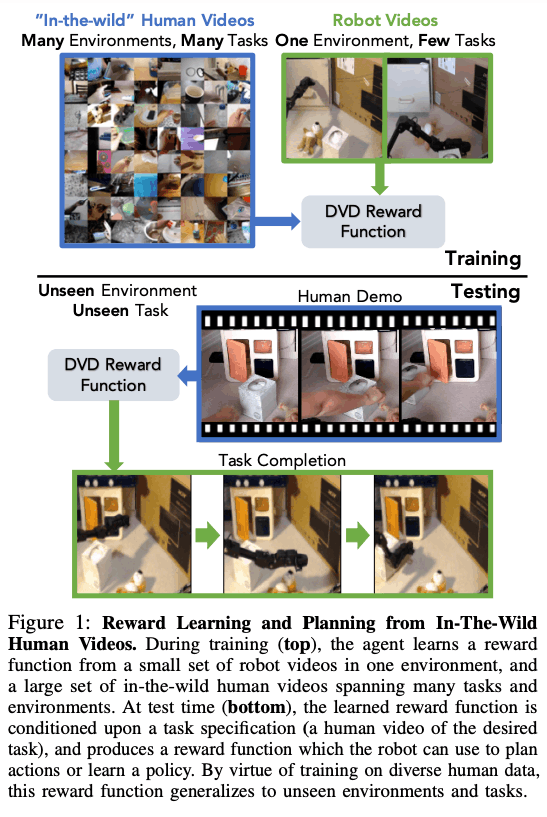In this section, we will go into further detail regarding the DVD methodology. The basic idea is to learn a reward function $\mathcal{R}_{\theta}$ that can capture the functional similarity (i.e., whether they are performing the same task) of two videos, one about task $\mathcal{T}_{i}$$and the other about task \mathcal{T}_{j}, when given two videos d_{i} and d_{j}. The idea is to learn a reward function \mathcal{R}_{\theta} that can Let \mathcal{D}^{h} and \mathcal{D}^{r} be the dataset of human videos and a small number of robot videos, respectively, and the two videos can be either video belonging to D^{h} or D^{r}. Then, as shown in the figure below, each video has an action label, which tells us which video belongs to which task.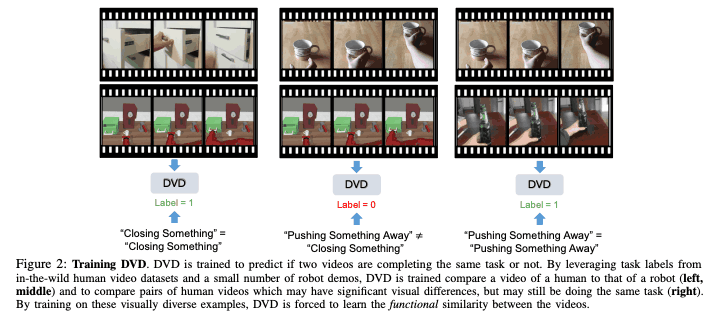The output of \mathcal{R}_{\theta} represents the similarity score at the task level of the two videos, and we learn to minimize the following objective function using AVERAGE cross-entropy loss to pairs of videos.$$\mathcal{J}(\theta)=\mathbb{E}_{\mathcal{D}^{h} \cup \mathcal{D}^{r}}[\log (\mathcal{R}_{\theta}(d_{i}, d_{i}^{\prime}))+\log (1-\ mathcal{R}_{\theta}(d_{i}, d_{j}))] $$### Regarding the implementation of DVD The reward function \mathcal{R}_{\theta} to be learned is$$ \mathcal{R}_{\theta}(d_{i}, d_{j}) = f_{sim}(f_{enc}(d_{i}), f_{enc}(d_{j}); \theta)$$. and let$h=f_{enc}$be the pre-trained video encoder, the fully connected neural network$f_{sim}(h_{i}, h_{j}; \theta)$represented by the parameter$\theta$is trained to estimate whether the video encoding$h_{i }$and$h_{j}$can be learned to estimate whether they are the same or not. Here, the video encoder$f_{enc}$drops the videos into the latent space, and the binary classifier$f_{sim}$receives those video encodings and is trained as in the previous section. Here, since there is a variation in the amount of robot and human videos, we try to balance them so that each batch$(d_{i}, d_{i}', d_{j})$has a 50% chance of being a robot video.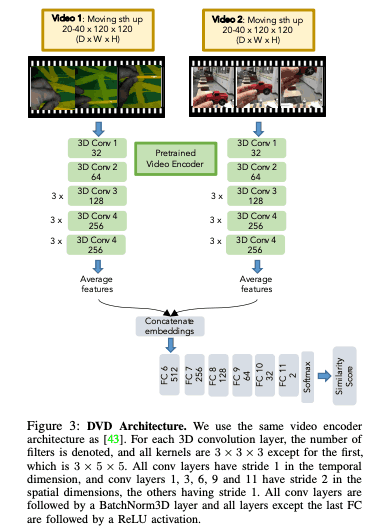### Performing Tasks with a DVD So how can we use the learned reward function$R_{\theta}$to solve the task? This reward function can be combined with either model-free RL or model-based RL. In this paper, we use a method called visual model predictive control, which uses a learned visual dynamics model to plan the robot's actions. At task execution time, a human demonstration$d_{i}$of the task to be solved is given, and the action is optimized so that the robot's behavior is similar to the demonstration. Now let's get a little more specific. First of all, we train an action-conditioned video prediction model$p_{\phi} (s_{t+1:t+H}|s_{t}, a_{t:t+H})$using SV2P model. Then, using the cross-entropy method (CEM) and the learned dynamics model$p_{\phi}$, we select an action to maximize the similarity with the given demonstration. In other words, the trajectories$\{s_{t+1:t+H}\}^{g}$of the action sequences of length H for G rollout are obtained as images through the learned dynamics model. Then, the trajectory with the maximum similarity is selected by comparing each trajectory with the demonstration.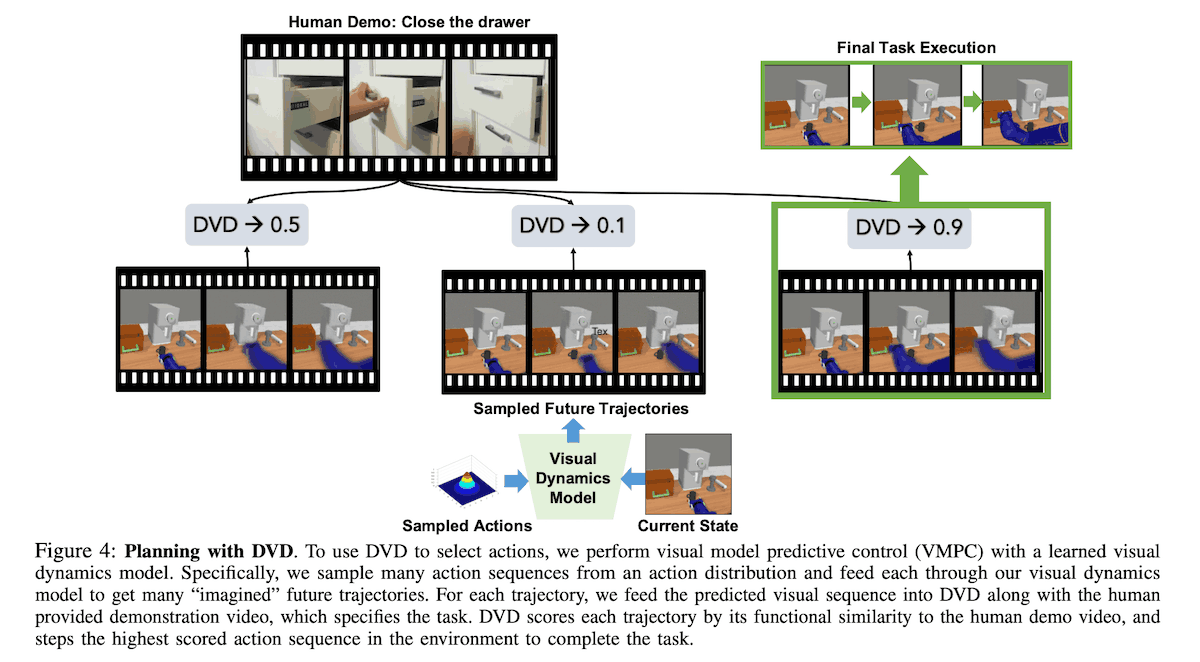## experiment In this paper, to investigate whether DVD effectively uses human video data to improve generalization performance, we conducted experiments that confirm that - Whether DVD generalizes to new environments - Whether DVDs generalize to novel tasks - Whether generalization by a single human demonstration of a DVD is more effective than other methods - Can reward be estimated for real robots? The first three are simulation tasks like the one on the left in the figure below, which are based on the learning environment, with the test environment Test Env1 having different colors, Test Env2 having different colors and viewpoints, and Test Env3 having different colors, viewpoints, and the order in which objects are lined up. We prepared three tasks: (1) closing the drawer, (2) turning the faucet to the right, and (3) pushing the cup from the camera to the coffee machine. We have conducted experiments using a human dataset called the Something-Something-V2 dataset, which contains a total of 174 classes and 220,837 videos. This dataset contains a wide variety of things that are manipulating objects by performing different actions on various environments and objects. In this experiment, we use videos of up to 15 different human tasks, with 853-3170 data for each task. In addition, we assumed that the robot was given 120 video demonstrations of three different tasks. For the experiments on the real machine, we used a WidowX200 robot to perform the experiments. Using the same setup as in the simulation, the DVD is learned by 80 robots and human videos in each of the two learning tasks in this experiment. The learning environment is an environment with a cupboard where the files are stored as shown in the right figure below. In the test environment, it is replaced by a toy kitchen set. The learning tasks are "Closing something" and "Pushing something left to right", and the test tasks are "Closing something" (known task) and "Pushing something right to left" (unknown task). The test task is "Closing something" (known task) and "Pushing something right to left" (unknown task).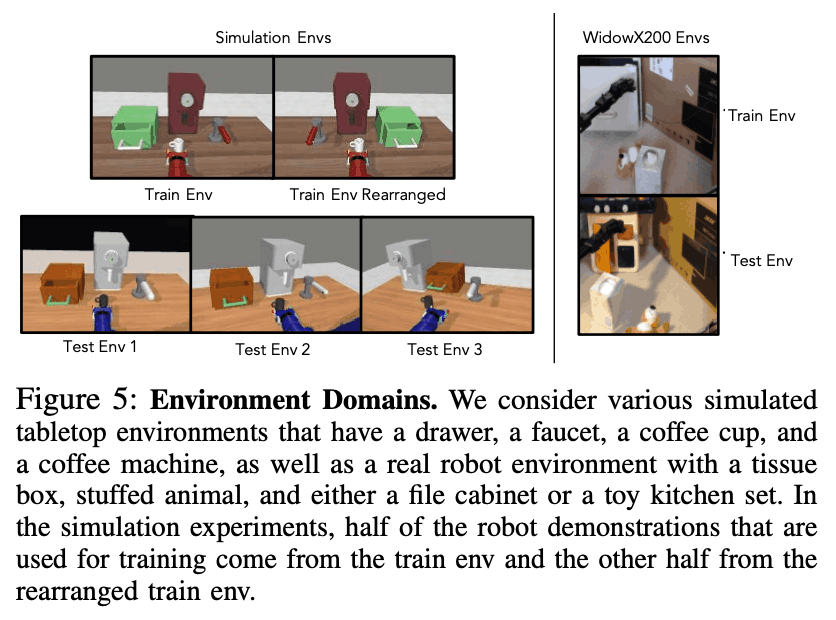When solving a task, a trajectory is selected that has similar behavior to the human video, given a video of an unknown human solving the task, as shown in the figure below.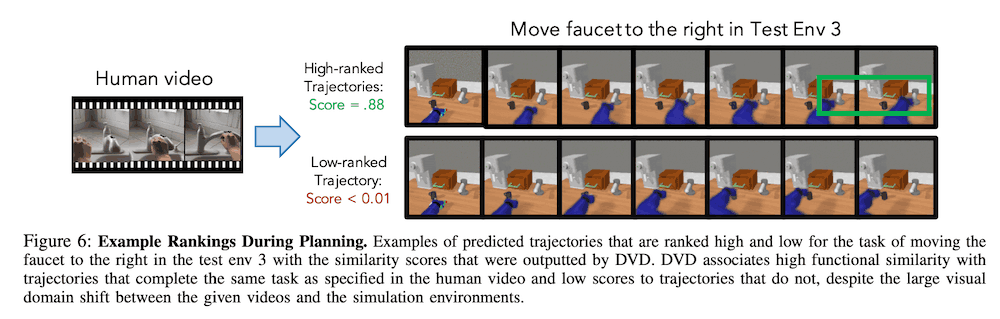### Generalization to new environments First, we investigate the generalization performance of the DVD to unknown environments. We examine the generalization performance against unknown environments by mixing different amounts of human data with the robot's videos in the training environment for the three target tasks. We hypothesize that using a variety of human data will result in a better performance against the unknown environment. To test this, we compare the performance of the robot-only (Robot Only) and the robot+K human tasks (Robot+K Human Tasks). Here, a$K$of 3 indicates that the data contains all of the data for the target task, while a$K$greater than 3 indicates that the data contains data for tasks unrelated to the target task. To evaluate these results, we examined the number of task successes by training the visual dynamics model and using the visual MPC and DVD output as rewards, as described in the previous section. data for training the visual dynamics model is automatically collected in the test environment. The data for training the visual dynamics model is automatically collected in the test environment. The following figure shows the results, and it can be seen that the number of successes is much higher when human data is included than when no human data is included, even if the data is not relevant to the task.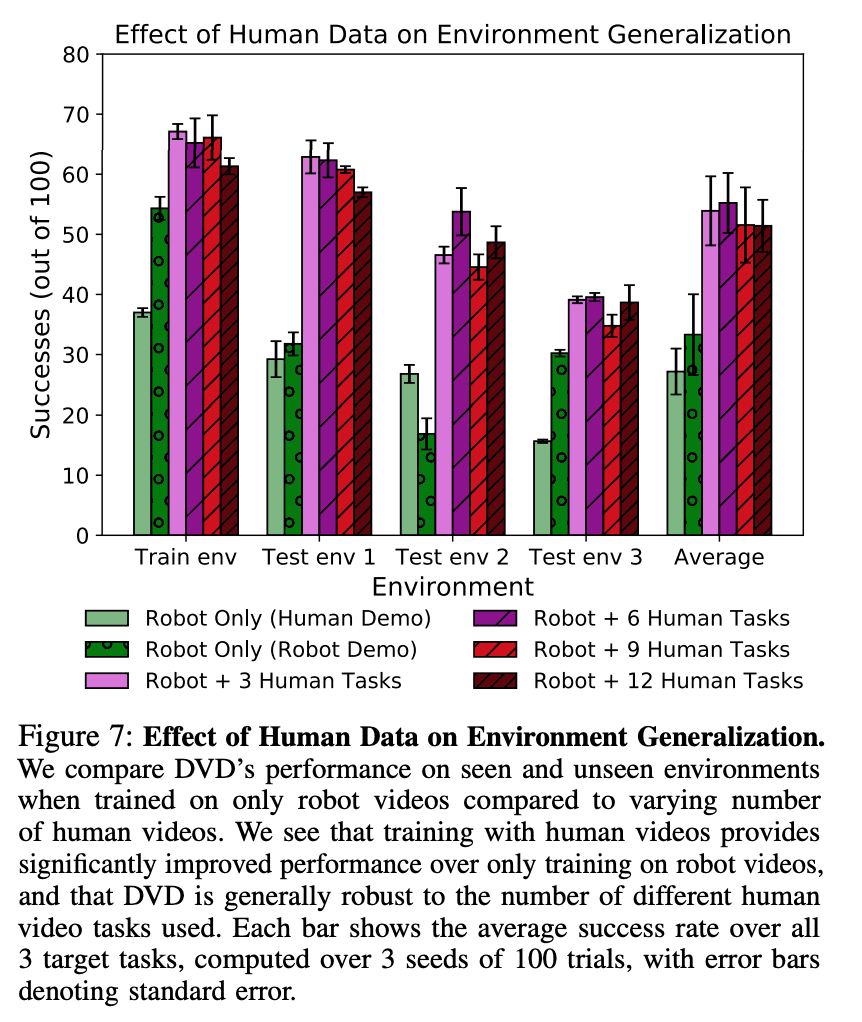### Generalization for unknown tasks Next, we experimented to test the generalization performance for an unknown task. Here, we did not include the data of the target task in training the DVD but used the robot's data and different amounts of human data for three tasks: (1) opening a drawer, (2) moving an object from right to left, and (3) not moving any object. As for the experimental environment, we wanted to investigate the generalization performance of the task, so we used the same training environment as the test environment. The table below shows the success rate of each task for each data case. From the results below, we can see that, as in the previous results, even if we add human data not related to the task, the success rate is higher on average when compared to the Robot Only case. In particular, in the case of Robot Only, the Move faucet to the right task is almost impossible to solve because it fails to identify the task itself.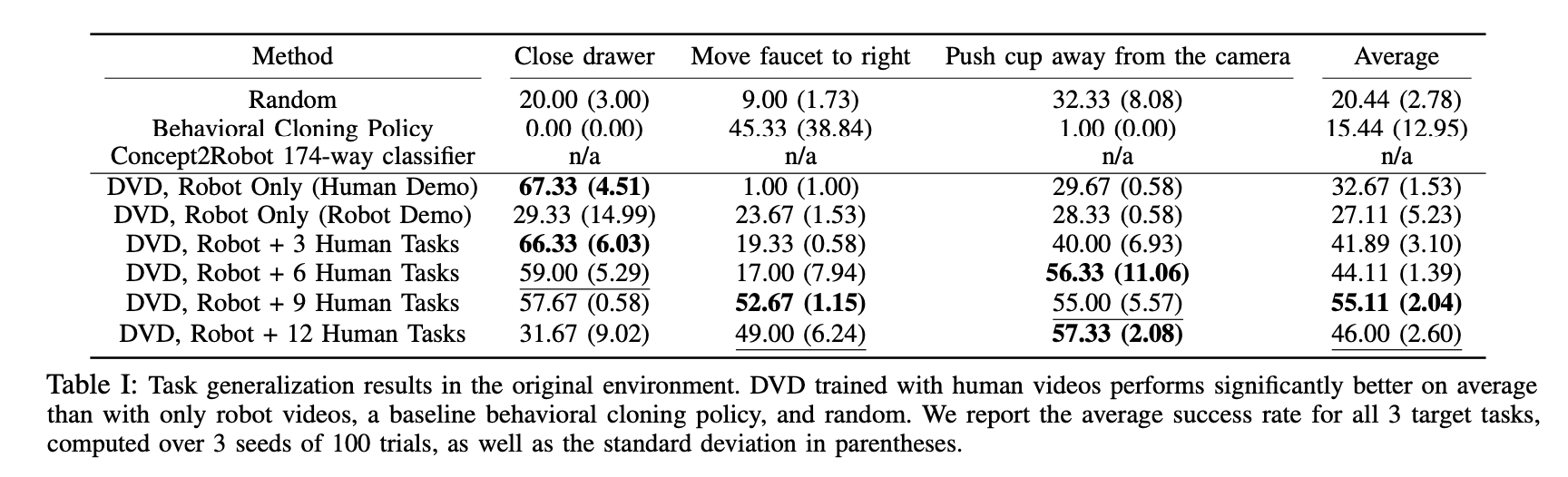### Comparison with other methods Here, we checked whether DVD can use human videos more effectively than other methods. One of our baselines is Concept2Robot, which uses the output of a video classifier trained on the Sth Sth V2 dataset as a reward. However, unlike DVD, Concept2Robot does not allow task execution conditional on demonstration, so we experimented with no condition. As another baseline, we used a method called demo-conditioned behavioral cloning, which is a method to learn actions by behavioral cloning conditioned on the robot or human demonstrations. In addition, we added random policy as a comparison target. The figure below shows the results, and it can be seen that DVD has the highest number of successes out of 100 trials compared to any other method. This is because Concept2Robot is unable to condition on demonstrations of unknown tasks to be solved.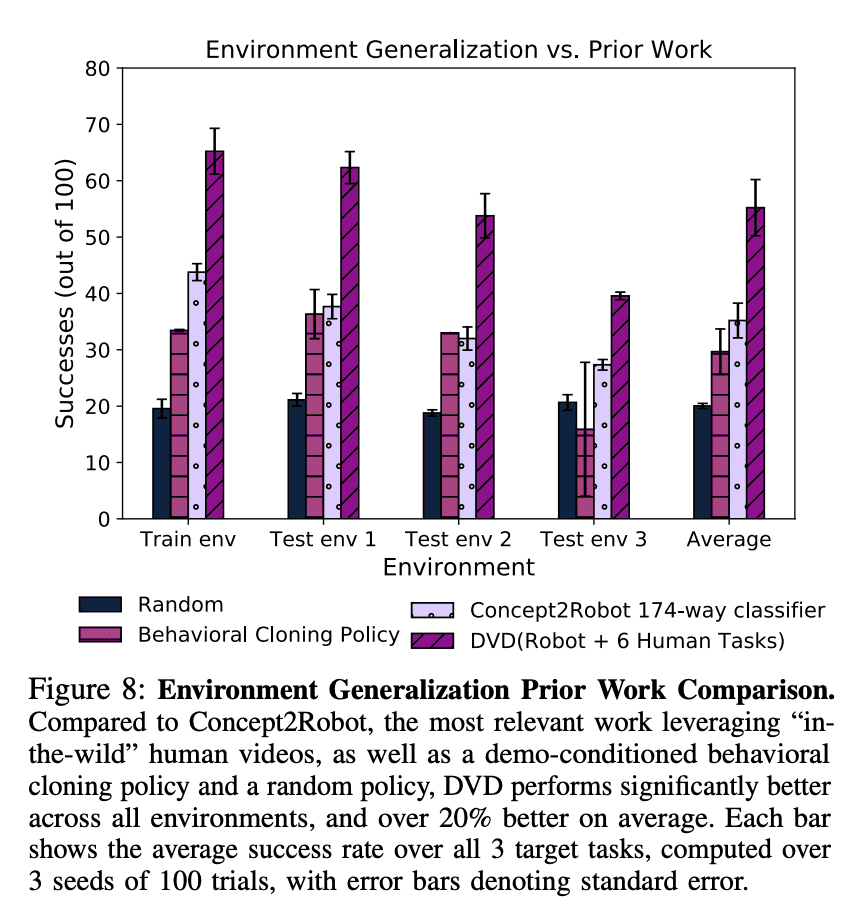### Effectiveness in practical experiments It worked well in the simulation experiment, but what about the actual experiment? In the real experiment, we compared the DVD with various amounts of human videos as before. The table below shows how many times we were able to solve the task out of 20 trials, and from the results below we can see that using human video is more successful than using only Robot data. The results below also show that using human data, which is irrelevant to the task ($K\$>3), results in more successes than not using human data.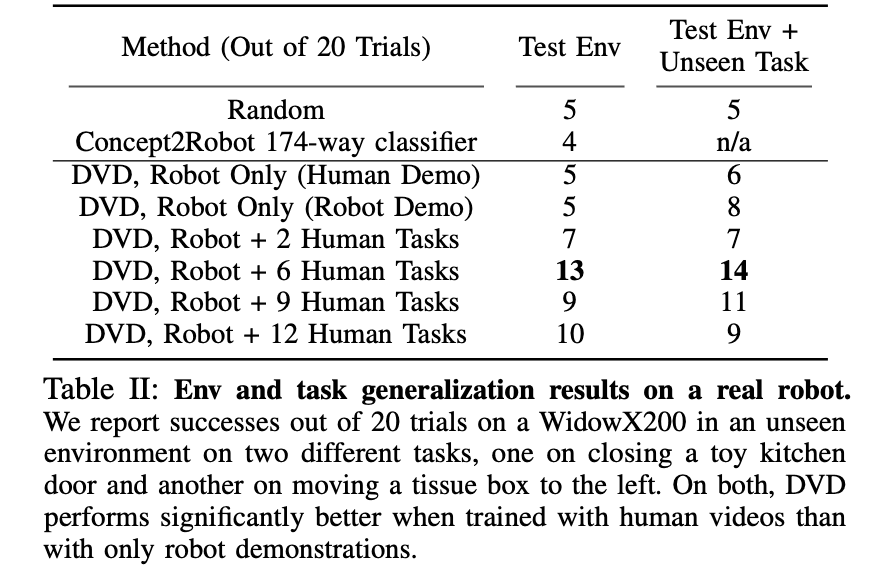### Relationship between the amount of data and performance of robots

Finally, we investigated the relationship between the amount of robot data and performance. In the previous experiments, we used 120 robot demonstrations, but the figure below shows the results of an experiment to see how performance would be affected if the amount of robot data were smaller. The figure below shows that there is no significant difference in the number of successful tasks even when the amount of data is reduced, indicating that even a small amount of DVD data from a robot can produce a good performance.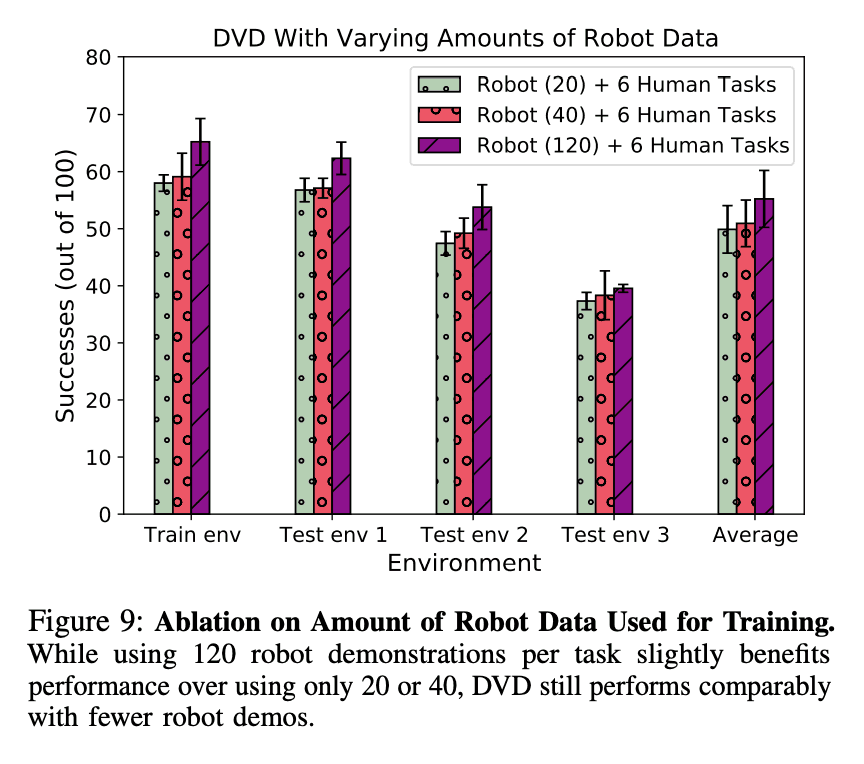## summary

The problem of difficulty in obtaining large-scale data for robots has been mentioned for a long time, and recently there has been a lot of research in the direction of improving learning efficiency and generalization performance, such as in this paper, by making good use of human data. However, since the domain difference between robot data and human data is too large, it is necessary to explore how to use robot data more effectively in the future.

If you have any suggestions for improvement of the content of the article,